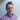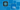Carl Rippon

# Repeating an element n times in React

August 11, 2020
react

In this post, we will cover how to output an element n times in JSX in React. This isn’t as straight forward as we might think …

## An example

Here’s a snippet of a component in React that needs to add a number of dynamic fields specified by the user:

``````const [
] = React.useState(1);
const [
] = React.useState(0);
return (
<div>
<div>
<input
type="number"
onChange={(e) =>
parseInt(e.currentTarget.value, 10)
)
}
/>
</div>
<button
onClick={() => {
);
}}
>
</button>
{/* TODO - add the dynamic fields */}
</div>
);``````

We also have a `Field` component:

``````const Field = ({ id }: { id: number }) => (
<div>
<label htmlFor={`field\${id}`}>Field {id}</label>
<input id={`field\${id}`} type="text" />
</div>
);``````

So, we need to render `Field`, a number of times defined by the user.

## A solution

We may think of using a `for` loop in JSX, because this is what we usually use when we need to do something n times:

``````{
for (
let i = 1;
i++
) {
<Field id={i} key={i} />;
}
}``````

… but this doesn’t work directly in JSX because the `for` loop doesn’t return anything to be added to the DOM.

We could use a `for` loop outside JSX to build the field elements and then output the field elements in JSX:

``````const fields: JSX.Element[] = [];for (let i = 1; i <= committedFieldsToAdd; i++) {  fields.push(<Field id={i} key={i} />);}
return (
<div>
...
<button
...
>
</button>
{fields}  </div>
);``````

This works, but there is a more concise solution.

## A more concise solution

Usually, we use the `map` method on an array when iterating in JSX. How can we create an array with the appropriate number of items that we can map over? Well, we can use the `Array` constructor passing in the number of elements to create:

``Array(committedFieldsToAdd);``

We can’t map over this though, because the elements are empty. So, a trick we can do is to spread the array into a new array:

``[...Array(committedFieldsToAdd)];``

This can then be mapped over to output the field as follows:

``````[
].map((value: undefined, index: number) => (
<Field id={index + 1} key={index} />
));``````

The first parameter in the `map` method is the value of the array element, which is `undefined`. The array index is the second parameter, which is more useful to us. So, we use the array index to form the React element key and the field id.

Neat!

A working example is available at the following CodeSandbox link:

https://codesandbox.io/s/repeat-element-dpocp?file=/src/App.tsx

## Did you find this post useful?

Let me know by sharing it on Twitter.